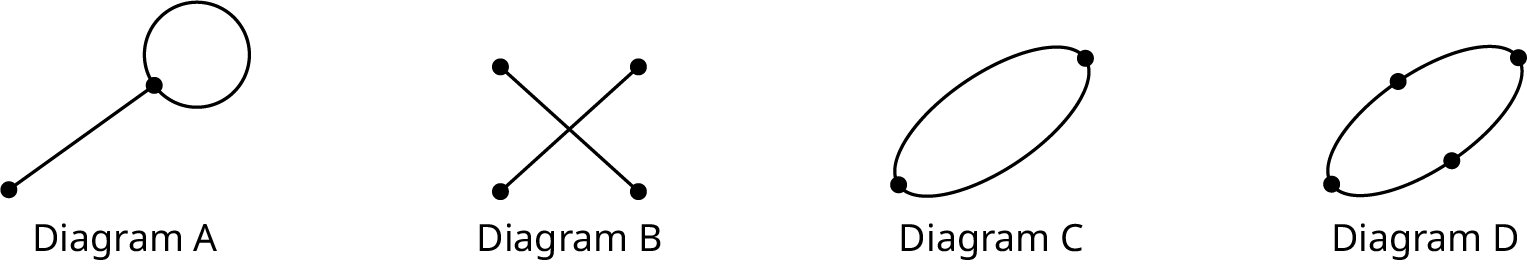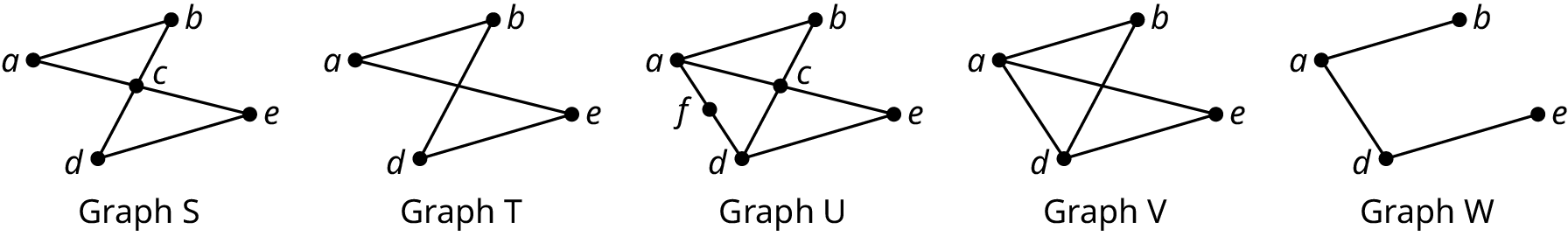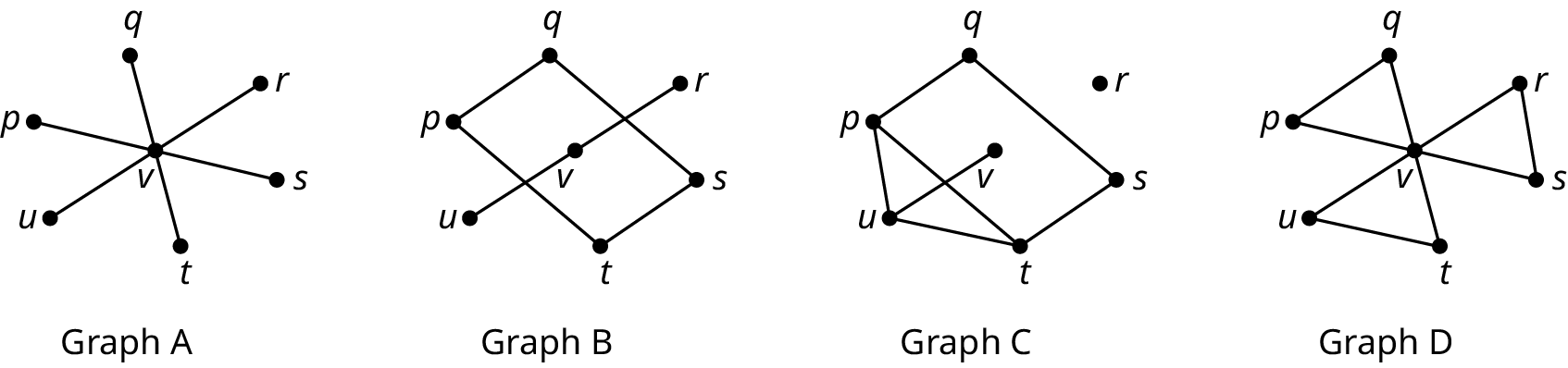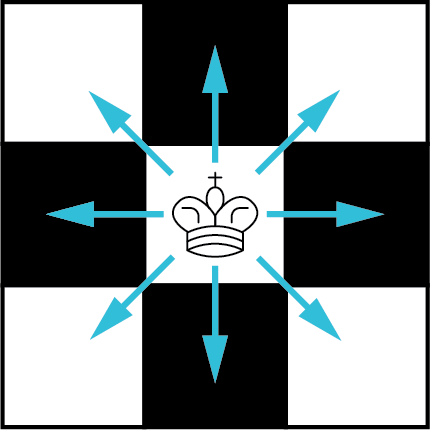Contemporary Mathematics

# 12.1Graph Basics

Contemporary Mathematics12.1 Graph Basics

Figure 12.2 Cell phone networks connect individuals. (credit: "Business people using their phones" by Rawpixel Ltd./Flickr, CC BY 2.0)

### Learning Objectives

After completing this section, you should be able to:

1. Identify parts of a graph.
2. Model applications of graph basics.

When you hear the word, graph, what comes to mind? You might think of the $xyxy$-coordinate system you learned about earlier in this course, or you might think of the line graphs and bar charts that are used to display data in news reports. The graphs we discuss in this chapter are probably very different from what you think of as a graph. They look like a bunch of dots connected by short line segments. The dots represent a group of objects and the line segments represent the connections, or relationships, between them. The objects might be bus stops, computers, Snapchat accounts, family members, or any other objects that have direct connections to each other. The connections can be physical or virtual, formal or casual, scientific or social. Regardless of the kind of connections, the purpose of the graph is to help us visualize the structure of the entire network to better understand the interactions of the objects within it.

### Parts of a Graph

In a graph, the objects are represented with dots and their connections are represented with lines like those in Figure 12.3. Figure 12.3 displays a simple graph labeled G and a multigraph labeled H. The dots are called vertices; an individual dot is a vertex, which is one object of a set of objects, some of which may be connected. We often label vertices with letters. For example, Graph G has vertices a, b, c, and d, and Multigraph H has vertices, e, f, g, and h. Each line segment or connection joining two vertices is referred to as an edge. H is considered a multigraph because it has a double edge between f and h, and a double edge between h and g. Another reason H is called a multigraph is that it has a loop connecting vertex e to itself; a loop is an edge that joins a vertex to itself. Loops and double edges are not allowed in a simple graph.

To sum up, a simple graph is a collection of vertices and any edges that may connect them, such that every edge connects two vertices with no loops and no two vertices are joined by more than one edge. A multigraph is a graph in which there may be loops or pairs of vertices that are joined by more than one edge. In this chapter, most of our work will be with simple graphs, which we will call graphs for convenience.

Figure 12.3 A Graph and a Multigraph

It is not necessary for the edges in a graph to be straight. In fact, you can draw an edge any way you want. In graph theory, the focus is on which vertices are connected, not how the connections are drawn (see Figure 12.4). In a graph, each edge can be named by the two letters of the associated vertices. The four edges in Graph X in Figure 12.4 are ab, ac, ad, and ae. The order of the letters is not important when you name the edge of a graph. For example, ab refers to the same edge as ba.

### Checkpoint

A graph may have vertices that are not joined to other vertices by edges, such as vertex f in Graph X in Figure 12.4, but any edge must have a vertex at each end.

Figure 12.4 Different Representations of the Same Graph

### Example 12.1

#### Identifying Edges and Vertices

Name all the vertices and edges of graph F in Figure 12.5.

Figure 12.5 Graph F

### Checkpoint

When listing the vertices and edges in a graph, work in alphabetical order to avoid accidentally listing the same item twice. When you are finished, count the number of vertices or edges you listed and compare that to the number of vertices or edges on the graph to ensure you didn’t miss any.

1.
Name all the vertices and edges of Graph A.
Figure 12.6 Graph A

Since the purpose of a graph is to represent the connections between objects, it is very important to know if two vertices share a common edge. The two vertices at either end of a given edge are referred to as neighboring, or adjacent. For example, in Figure 12.5, vertices x and w are adjacent, but vertices y and w are not.

### Example 12.2

#### Identifying Vertices That Are Not Adjacent

Name all the pairs of vertices of graph F in Figure 12.5 that are not adjacent.

1.
Name all the pairs of vertices of graph A in Figure 12.6 that are not adjacent.

### People in Mathematics

#### Sergey Brin and Laurence Page

The “Google boys,” Sergey Brin and Laurence Page, transformed the World Wide Web in 1998 when they used the mathematics of graph theory to create an algorithm called Page Rank, which is known as the Google Search Engine today. The two computer scientists identified webpages as vertices and hyperlinks on those pages as edges because hyperlinks connect one website to the next. The number of edges influences the ranking of a website on the Google Search Engine because the websites with more links to “credible sources” are ranked higher. ("Page Rank: The Graph Theory-based Backbone of Google," September 20, 2011, Cornell University, Networks Blog.

### Analyzing Geographical Maps with Graphs

Figure 12.7 Commercial airlines' route systems create a global network.

When graphs are used to model and analyze real-world applications, the number of edges that meet at a particular vertex is important. For example, a graph may represent the direct flight connections for a particular airport as in Figure 12.8. Representing the connections with a graph rather than a map shifts the focus away from the relative positions and toward which airports are connected. In Figure 12.8, the vertices are the airports, and the edges are the direct flight paths. The number of flight connections between a particular airport and other South Florida airports is the number of edges meeting at a particular vertex. For example, Key West has direct flights to three of the five airports on the graph. In graph theory terms, we would say that vertex FYW has degree 3. The degree of a vertex is the number of edges that connect to that vertex.

Figure 12.8 Direct Flights between South Florida Airports

### Example 12.3

#### Determining the Degree of a Vertex

Determine the degree of each vertex of Graph J in Figure 12.8. If graph J represents direct flights between a set of airports, do any of the airports have direct flights to two or more of the other cities on the graph?

Figure 12.9 Graph J

1.
Name a vertex of Graph A in Figure 12.6 with degree 4.

Graphs are also used to analyze regional boundaries. The states of Utah, Colorado, Arizona, and New Mexico all meet at a single point known as the “Four Corners,” which is shown in the map in Figure 12.11.

Figure 12.11 Map of the Four Corners

In Figure 12.12, each vertex represents one of these states, and each edge represents a shared border. States like Utah and New Mexico that meet at only a single point are not considered to have a shared border. By representing this map as a graph, where the connections are shared borders, we shift our perspective from physical attributes such as shape, size and distance, toward the existence of the relationship of having a shared boundary.

Figure 12.12 Graph of the Shared Boundaries of Four Corners States

### Example 12.4

#### Graphing the Midwestern States

A map of the Midwest is given in Figure 12.13. Create a graph of the region in which each vertex represents a state and each edge represents a shared border.

Figure 12.13 Map of Midwestern States

1.
The figure shows a map of the Island of Oahu in the State of Hawaii divided into regions. Draw a graph in which each vertex represents one of the regions and each edge represents a shared land border.
Figure 12.17 Map of Oahu

### Graphs of Social Interactions

Geographical maps are just one of many real-world scenarios which graphs can depict. Any scenario in which objects are connected to each other can be represented with a graph, and the connections don’t have to be physical. Just think about all the connections you have to people around the world through social media! Who is in your network of Twitter followers? Whose Snapchat network are you connected to?

### Example 12.5

#### Graphing Chloe’s Roblox Friends

Roblox is an online gaming platform. Chloe is interested to know how many people in her network of Roblox friends are also friends with each other so she polls them. Explain how a graph or multigraph might be drawn to model this scenario by identifying the objects that could be represented by vertices and the connections that could be represented by edges. Indicate whether a graph or a multigraph would be a better model.

1.
In a particular poker tournament, five groups of five players will play at a table until one player wins, then the five winning players will play each other at a table in a final round. Explain how a graph or multigraph might be drawn to model this scenario by identifying the objects that could be represented by vertices and the connections that could be represented by edges. Indicate whether a graph or a multigraph would be a better model.

### Who Knew?

#### Using Graph Theory to Reduce Internet Fraud

Could graphs be used to reduce Internet fraud? At least one researcher thinks so. Graph theory is used every day to analyze our behavior, particularly on social network sites. Alex Buetel, a computer scientist from Carnegie Mellon University in Pittsburgh, Pennsylvania, published a research paper in 2016 that discussed the possibilities of distinguishing the normal interactions from those that might be fraudulent using graph theory. Buetel wrote, “To more effectively model and detect abnormal behavior, we model how fraudsters work, catching previously undetected fraud on Facebook, Twitter, and Tencent Weibo and improving classification accuracy by up to 68%.” In the same paper, the researcher discusses how similar techniques can be used to model many other applications and even, “predict why you like a particular movie.” (Alex Beutel, "User Behavior Modeling with Large-Scale Graph Analysis," http://reports-archive.adm.cs.cmu.edu/anon/2016/CMU-CS-16-105.pdf, May 2016, CMU-CS-16-105, Computer Science Department, School of Computer Science, Carnegie Mellon University, Pittsburgh, PA)

1.
A simple graph has no loops.
1. True
2. False
2.
It is not possible to have a vertex of degree 0.
1. True
2. False
3.
A graph with three vertices has at most three edges.
1. True
2. False
4.
A multigraph with three vertices has at most three edges.
1. True
2. False
5.
If vertex a is adjacent to vertex b, and vertex b is adjacent to vertex c, then vertex a must be adjacent to vertex c.
1. True
2. False

### Section 12.1 Exercises

For the following exercises, use the figure showing Diagrams A, B, C, and D.1.
Identify any multigraphs.
2.
Identify any graphs.
3.
Identify any multigraph that has a loop.
4.
Identify any multigraph that has a double edge.
For the following exercises, use the figure showing Graphs S, T, U, V, and W.5.
Determine the number of vertices in Graph T.
6.
Determine the number of vertices in Graph U.
7.
Identify the graph with the most vertices.
8.
Identify the graphs with four vertices.
9.
Identify the graph with the most edges.
10.
Identify the graph with the fewest edges.
11.
Name the vertices in Graph S.
12.
Name the vertices in Graph V.
13.
Determine the number of edges in Graph U.
14.
Determine the number of edges in Graph T.
15.
Name the edges in Graph V.
16.
Name the edges in Graph W.
17.
Identify any pairs of vertices in Graph T that are not adjacent.
18.
Identify any vertices in Graph V that are not adjacent.
19.
Find the degree of vertex a in graph U.
20.
Find the degree of vertex a in graph S.
21.
Which two graphs have a vertex of degree 4?
For the following exercises, use the figure showing Graphs A, B, C, and D.22.
Identify the graph with 1 vertex of degree 6 and 6 vertices of degree 1.
23.
Identify the graph with 1 vertex of degree 6 and 6 vertices of degree 2.
24.
Identify the graph with exactly 1 vertex of degree 0.
25.
Identify the graph with exactly 2 vertices of degree 1.
26.
Name all the pairs of adjacent vertices in Graph B.
27.
Name all the pairs of adjacent vertices in Graph A.
28.
Identify the graph in which the sum of the degrees of the vertices is 14.
For each of the following exercises, a scenario is given. Explain how a graph or multigraph might be drawn to model the scenario by identifying the objects that could be represented by vertices and the connections that could be represented by edges. Indicate whether a graph or a multigraph would be a better model.
29.
The Centers for Disease Control and Prevention (CDC) is tracking the spread of a virus. The CDC is attempting to determine where the virus began by identifying where each known carrier contracted the virus.
30.
The faculty members in a college mathematics department have a “telephone tree” that assigns each faculty member two other faculty members to call with information in the event of an emergency. The chairperson of the department contacts two faculty members. Each of these faculty members contacts two faculty members, and so on, until all faculty members have been notified.
31.
There is a theory that any two people on earth are no more than six social connections apart, which is known as “six degrees of separation” or the “six handshake rule.” It means that there is a chain of a “friend of a friend” that connects any two people through at most six steps.
32.
The U.S. Postal Service has a network of post offices that move mail around the United States. Mail trucks from one post office follow routes deliver mail to other locations. These mail trucks also pick up mail to bring back to their home post office.
In the following exercises , draw a graph to represent the given scenario.
33.
Draw a graph to model the scenario described in Your Turn 12.5.
34.
In the game of chess, a king can move one space in any direction, vertically, horizontally, or diagonally, as indicated in the figure. Draw a graph to represent the possible movements of a king on a three-by-three section of a chess board such that each edge represents one move, and each vertex represents a position where the king might be at the beginning or end of a turn. Assume that the king can have unlimited turns.35.
Chloe is interested to know how many in her network of Roblox friends are also friends with each other, so she polls them. The following is a list of each of Chloe’s friends and their common friends. Create a graph of the Roblox friends in Chloe’s network, including Chloe.
• Aria is friends with no one else in Chloe’s network.
• Benicio is friends with Dakshayani, Eun-ah, and Fukashi.
• Dakshayani is friends with Benicio.
• Eun-ah is friends with Benicio and Fukashi.
• Fukashi is friends with Benicio and Eun-ah.